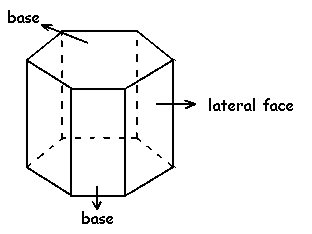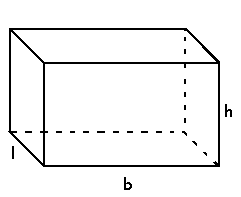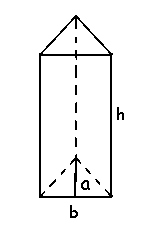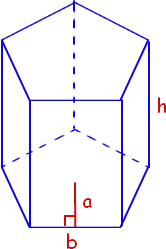# Prism Formula

A polyhedron with two polygonal bases parallel to each other is a prism. In optics, the prism is the transparent optical element with flat polished surfaces that refract light. The two polygonal bases are joined by lateral faces. The lateral faces are mostly rectangle. It may be a parallelogram in some cases.The Prism Formulas, in general, are given as,

Surface area of a prism = 2 × Base area  + Lateral surface area

Volume = Base area × Height

Prisms are of different types. They are named based on the base shape of a prism. The types of prisms are rectangular prism, triangular prism, pentagonal prism and hexagonal prism.

Rectangular prism: A Prism that has 2 parallel rectangular bases and 4 rectangular faces is a Rectangular Prism.The Rectangular Prism Formulas are,

Base area of a Rectangular prism = bl

Surface area of a Rectangular prism = 2(bl + lh + hb)

Volume of a Rectangular prism = lbh

Where,
b – base length of the rectangular prism
l – base width of the rectangular prism
h – the height of the rectangular prism

Triangular Prism: A prism that has 3 rectangular faces and 2 parallel triangular bases, then it is a triangular prism.Base area of a Triangular prism = (1/2) × ab

Surface area of a Triangular prism = ab + 3bh

Volume of a Triangular prism = (1/2) × abh

Where,
a – apothem length of the triangular prism
b – base length of the triangular prism
h – the height of the triangular prism

Pentagonal Prism: A prism that has 5 rectangular faces and 2 parallel pentagonal bases is a pentagonal prism:The Pentagonal Prism Formulas are,

Base area of Pentagonal prism = (5/2) × ab

Surface area of a Pentagonal prism = 5ab + 5bh

Volume of a Pentagonal prism = (5/2) × abh

Where,
a – apothem length of the pentagonal prism
b – base length of the pentagonal prism
h – the height of the pentagonal prism

Hexagonal Prism: A hexagonal prism is a type of prism that has six rectangular faces and two parallel hexagonal bases.The Hexagonal Prism Formulas are,

Base area of Hexagonal prism = 3ab

Surface area of Hexagonal prism = 6ab + 6bh

Volume of Hexagonal prism = 3abh

Where,
a – apothem length of the hexagonal prism
b – base length of the hexagonal prism
h – the height of the hexagonal prism

 More topics in Prism Formulas Surface Area of a Prism Formula Volume of a Triangular Prism Formula Volume of a Rectangular Prism Formula